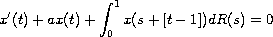Electron. J. Diff. Eqns., Vol. 2008(2008), No. 112, pp. 1-16.

### On stability and oscillation of equations with a distributed delay which can be reduced to difference equations Elena Braverman, Sergey Zhukovskiy

Abstract:
For the equation with a distributed delaywe obtain necessary and sufficient conditions of stability, exponential stability and oscillation. These results are applied to some particular cases, such as integro-differential equations and equations with a piecewise constant argument. Well known results for equations with a piecewise constant argument are obtained as special cases.

Submitted April 26, 2008. Published August 15, 2008.
Math Subject Classifications: 34K20, 34K11, 34K06, 39A11.
Key Words: Piecewise constant arguments; distributed delay; difference equations; oscillation; stability; exponential stability; integro-differential equations.

Show me the PDF file (336 KB), TEX file, and other files for this article.Elena Braverman Department of Mathematics and Statistics, University of Calgary 2500 University Drive N.W., Calgary, AB, Canada T2N 1N4 email: maelena@math.ucalgary.ca  Fax (403)-282-5150  Phone (403)-220-3956 Sergey Zhukovskiy Department of Mathematics and Statistics, University of Calgary 2500 University Drive N.W., Calgary, AB, Canada T2N 1N4 email:sergey@math.ucalgary.ca, s-e-zhuk@yandex.ru